Using the Euler equations for two dimensional motion

The basic equations for two-dimensional motion are the two scalar equations obtained from writing Euler’s first law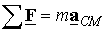plus one of the following three forms of Euler’s second law

1.      If the body is pinned at O to an inertial frame then use the table and/or the parallel axis theorem (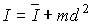) to find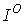and write: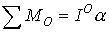2.     Or you can always take moments about the center of mass using the equation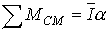3.     Or you can always take moments about any arbitrary point P using the equation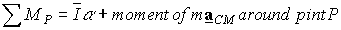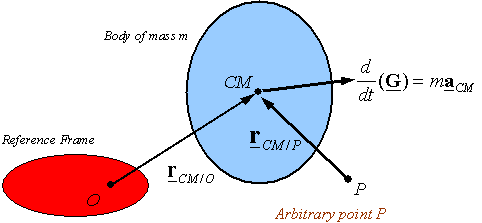Copy and distribute freely for personal use only

Department of Engineering Mechanics, University of Nebraska, Lincoln, NE 68588-0526

Last updated: 3/9/02 7:39:03 PM